## Given problem

Given a tree that looks like an below image.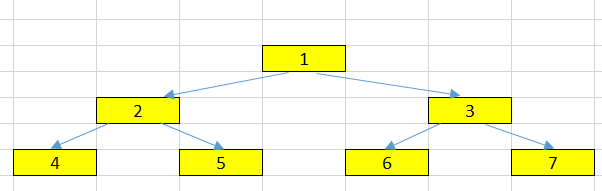• Node and some things around it.
• Degree
• Edge
• Path
• Distance
• Depth
• Level
• Height
• Width

## Node of a Tree

A node is a structure which may contain a value or condition, or represent a separate data structure.

In an above image, we can have some nodes: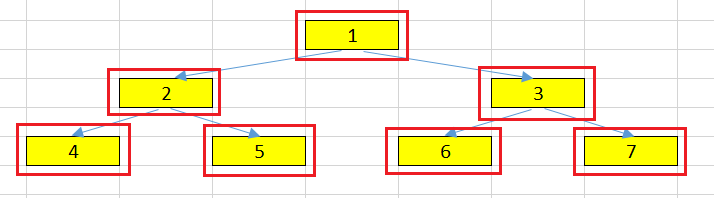Normally, in binary tree, we can define a node like that.

``````class TreeNode {
int value;
TreeNode left;
TreeNode right;
}
``````

Belows are some concepts that are relevants to a Node.

1. Root node

The top node in a tree, the prime ancestor.

For example, our root node in an above tree is `1`.

2. Child node

A node directly connected to another node when moving away from the root, an immediate descendant.

For example, `2` and `3` are the child nodes of the `root node` - `1`.

3. Parent node

The converse notion of a child, an immediate ancestor.

For example, 1 is the parent node of two child nodes: 2 and 3.

4. Sibling nodes

A group of nodes with the same parent.

For example, 2 and 3 are the sibling nodes. They have the same parent node - 1.

5. Descendant node

A node reachable by repeated proceeding from parent to child. Also known as subchild.

For example, 3, 7, and 6 are descendant nodes of 1.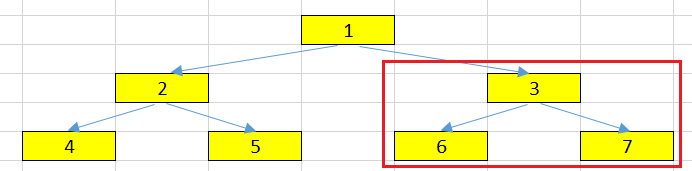6. Ancestor node

A node u is an ancestor of a node v if v is contained in the subtree rooted at u; we may write equivalently that v is a descendant of u.

For example, 1 is an ancestor node for every node such as 2, 3, 4, 5, 6, and 7.

7. Leaf node

A node with no children.

For example, 4, 5, 6 and 7 are leaf nodes in our tree.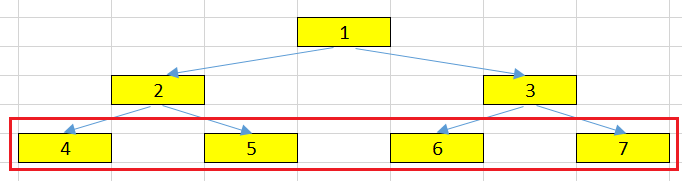## Degree of a Node

For a given node, it’s the number of children. A leaf is necessarily degree zero.

The degree of a tree is the maximum degree of a node.

For example:

• the degree of node 1 is 2 because it has two nodes: 2 and 3.
• the degree of an above tree is 2.

## Edge, Path, and Distance

1. Edge

Edge is the connection between one node and another.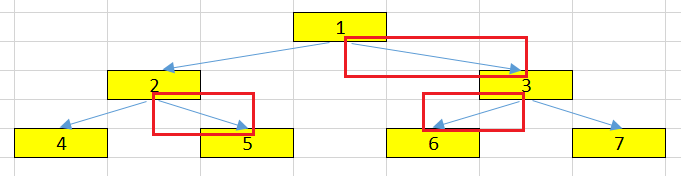2. Path

Path is a sequence of nodes and edges connecting a node with a descendant.

Path includes all nodes and all edges along the path, not just edges.

For example, the path between two nodes 4 and 5 is: 4 –> 2 –> 5.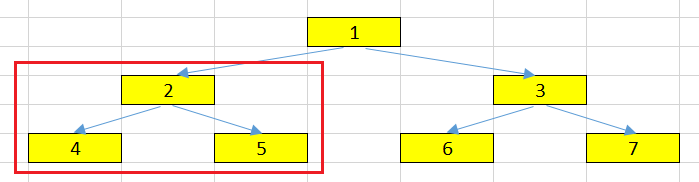3. Distance

The number of edges along the shortest path between two nodes.

For example, the distance between 4 and 5 is 2.## Depth, Level, Height, and Width

1. Depth

The distance between a node and the root.

The depth of the root node is 0.

For example, the depth of 5 node is 2.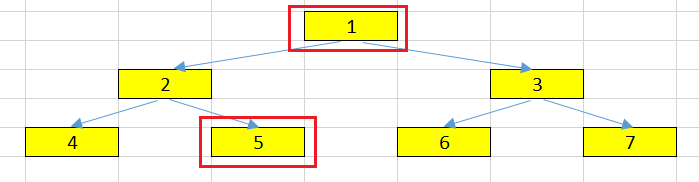2. Level

The level of a node is defined by 1 + the number of edges between the node and the root.

Below is the relationship between Level and Depth concepts.

`````` Level = Depth + 1
``````

The level of root node is 1.

For example, the level of 6 node in an above tree is 3.

Below is the source code that calculates the maximum level of binary tree.

We use top-down recursive version for this problem because:

• the starting point is the root node.
• we know that the level of root node is 1.
`````` public class MaxLevelTree {

private static int maxHeight = Integer.MIN_VALUE;

private static void getMaxLevelTopDown(TreeNode root, int level) {
if (root == null) {
return;
}

if (root.left == null && root.right == null) {
maxHeight = Math.max(maxHeight, level);
}

getMaxLevelTopDown(root.left, level + 1);
getMaxLevelTopDown(root.right, level + 1);
}
}
``````
3. Height

The height of a node is the number of edges on the longest downward path between that node and a leaf.

For example:

• the height of root node is 2.
• the height of 2 node is 1.

Below is the source code to calculate the height of binary tree. We will use bottom-up recursive version because:

• if the node is null, then its height is -1.
• the leaf node’s height is 0.
• It means that we have sufficient information about the height of the children nodes.
`````` public static int getHeightTree(TreeNode root) {
if (root == null) {
return -1;
}

int leftHeight = getMaxLevelBottomUp(root.left);
int rightHeight = getMaxLevelBottomUp(root.right);

return Math.max(leftHeight, rightHeight) + 1;
}
``````
4. Width

The number of nodes in a level.

For example:

• at the level = 2, we have two nodes such as 2 and 3.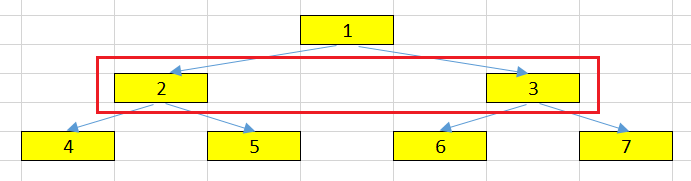• at the level = 3, we have four nodes.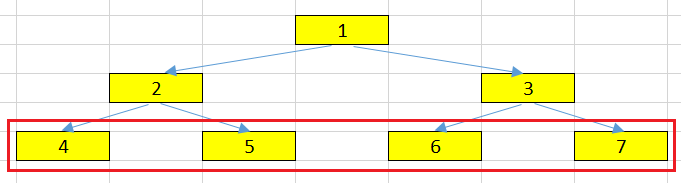## Wrapping up

• With depth and level concept, remember that it compares with the root node.

• With height concept, it compares with the leaf node.

Refer:

https://en.wikipedia.org/wiki/Tree_%28data_structure%29

https://www.cs.yale.edu/homes/aspnes/pinewiki/BinaryTrees.html?highlight=%28CategoryAlgorithmNotes%29